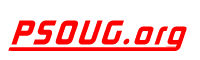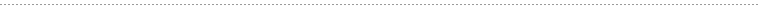Quick Search:PHP Code: Calculate the difference between two times formatted in HH:MM Jump to: Select a Location PSOUG Home Page Oracle Code Library mySQL Code Library PHP Code Library JavaScript Code Library Oracle Terms & Definitions Oracle Error Codes Advanced Code Search Contact UsSnippet Name: Calculate the difference between two times formatted in HH:MM

Description: A script that calculates the difference between two times, formatted in HH:MM. For example, if you enter 23:40 and 01:30, it will calculate it as a time difference of "01:50".

Comment: (none)

Language: PHP
Highlight Mode: PHP

```<?PHP

ECHO getTimeDiff("23:30","01:30");

FUNCTION getTimeDiff(\$dtime,\$atime){

\$nextDay=\$dtime>\$atime?1:0;
\$dep=EXPLODE(':',\$dtime);
\$arr=EXPLODE(':',\$atime);
\$diff=ABS(MKTIME(\$dep,\$dep,0,DATE('n'),DATE('j'),DATE('y'))-MKTIME(\$arr,\$arr,0,DATE('n'),DATE('j')+\$nextDay,DATE('y')));
\$hours=FLOOR(\$diff/(60*60));
\$mins=FLOOR((\$diff-(\$hours*60*60))/(60));
\$secs=FLOOR((\$diff-((\$hours*60*60)+(\$mins*60))));
IF(STRLEN(\$hours)<2){\$hours="0".\$hours;}
IF(STRLEN(\$mins)<2){\$mins="0".\$mins;}
IF(STRLEN(\$secs)<2){\$secs="0".\$secs;}
RETURN \$hours.':'.\$mins.':'.\$secs;
}

?> ```